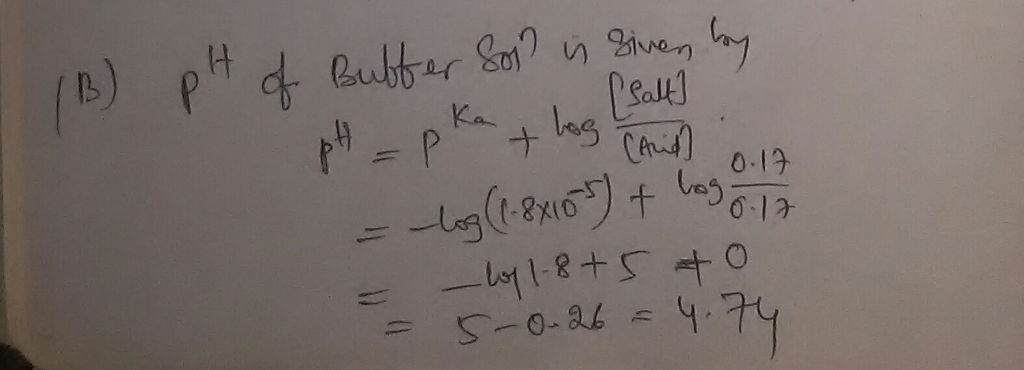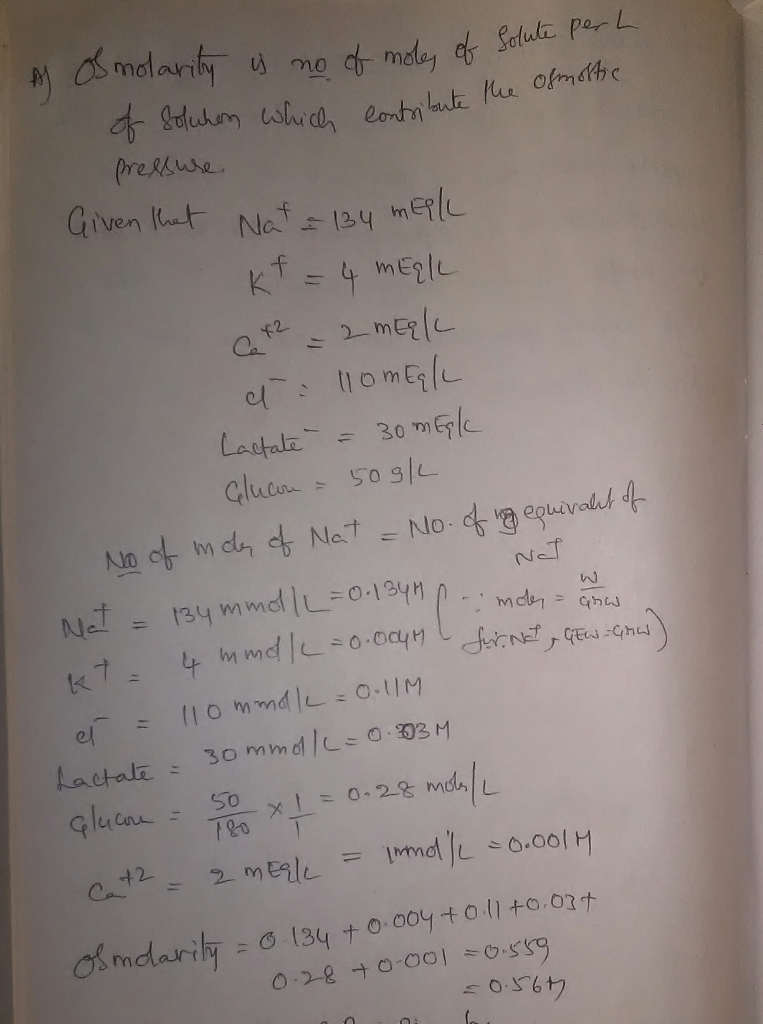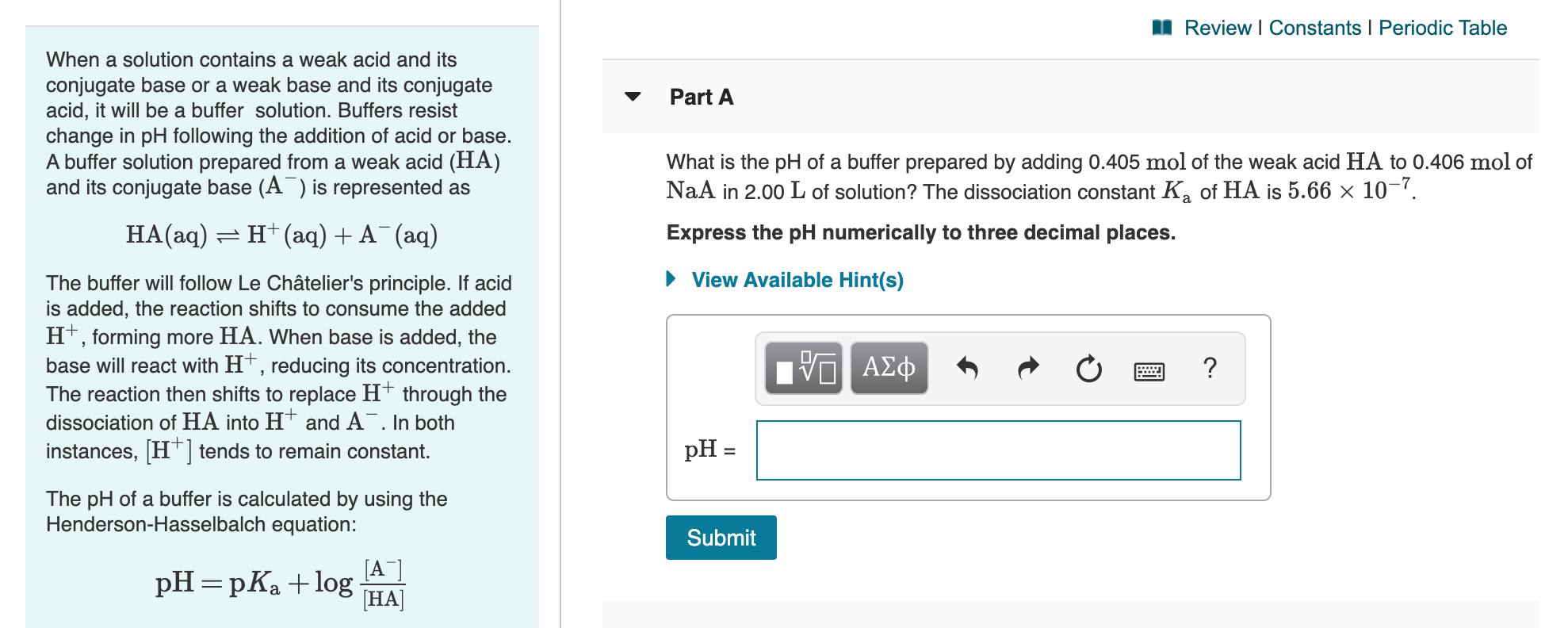# Part A. A lactated Ringer's solution with 5% glucose contains Na+ 134 mEq/L , K+ 4 mEq/L , Ca2+ 2...

Part A. A lactated Ringer's solution with 5% glucose contains Na+ 134 mEq/L , K+ 4 mEq/L , Ca2+ 2 mEq/L , Cl− 110. mEq/L , lactate− 30. mEq/L , and 50. g/L glucose (C6H12O6). What is the osmolarity (Osm) of the solution? Express the osmolarity to two decimal places.

Part B: Acetic acid has a Ka of 1.8×10−5. What is the pH of a buffer solution containing 0.17 M HC2H3O2 (acetic acid) and 0.17 M C2H3O2−? Express your answer using two decimal places.

Part B:

PH of the buffer solution is given by Henderson's equation.

Given that concentration of acid = 0.17M

Concentration of acetate ion = 0.17M##### Add Answer of: Part A. A lactated Ringer's solution with 5% glucose contains Na+ 134 mEq/L , K+ 4 mEq/L , Ca2+ 2...
Similar Homework Help Questions
• ### Each of the following mixtures is placed in a dialyzing bag and immersed in distilled water. Whic...

Each of the following mixtures is placed in a dialyzing bag and immersed in distilled water. Which substances will be found outside the bag in the distilled water? starch solution (colloid) and alanine, an amino acid, solution starch solution (colloid) and alanine, an amino acid, solution starch alanine starch and alanine none of the above NaCl solution and starch solution (colloid) solution and starch solution (colloid) starch Na+ and Cl− ions starch and Na+ and Cl− ions none of the...

• ### A 1.0-L buffer solution contains 0.100 mol﻿ HC2H3O2 and 0.100 mol﻿ NaC2H3O2. The value of Ka for HC2H3O2 is 1.8×10−5. Ca...

A 1.0-L buffer solution contains 0.100 mol﻿ HC2H3O2 and 0.100 mol﻿ NaC2H3O2. The value of Ka for HC2H3O2 is 1.8×10−5. Calculate the pH of the solution, upon addition of 0.070 mol of NaOH to the original buffer. Express your answer using two decimal places.

• ### A 1.0L buffer solution contains 0.100 molHC2H3O2 and 0.100 molNaC2H3O2. The value of Ka for HC2H3O2 is 1.8×10−5. Part A...

A 1.0L buffer solution contains 0.100 molHC2H3O2 and 0.100 molNaC2H3O2. The value of Ka for HC2H3O2 is 1.8×10−5. Part A Calculate the pH of the solution upon the addition of 0.015 mol of NaOH to the original buffer. Express your answer to two decimal places. Part B Calculate the pH of the solution upon the addition of 10.0 mL of 1.00 MHCl to the original buffer. Express the answer to two decimal places.

• ### M Review | Constants | Periodic Table - Part A When a solution contains a weak...M Review | Constants | Periodic Table - Part A When a solution contains a weak acid and its conjugate base or a weak base and its conjugate acid, it will be a buffer solution. Buffers resist change in pH following the addition of acid or base. A buffer solution prepared from a weak acid (HA) and its conjugate base (A) is represented as What is the pH of a buffer prepared by adding 0.405 mol of the weak acid...

Need Online Homework Help?